# 5.3序列模型与注意力机制

### 觉得有用的话,欢迎一起讨论相互学习~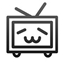• 对于机器翻译来说，给定输入的句子，会返回一个随机的英语翻译结果，但是你想要一个最好的英语翻译结果。对于语音识别也是一样，给定一个输入的语音片段，你不会想要一个随机的文本翻译结果，你想要一个最接近愿意的翻译结果。
• Jane visite l'Afrique en septembre 希望翻译成英文句子 Jane is visiting Africa in September
1. 集束搜索第一步就是挑选出英文翻译句子中的第一个单词。

1. 首先英语字典可表示为：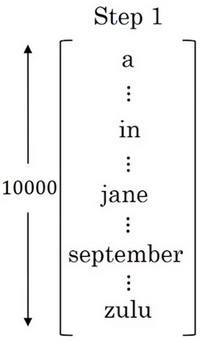2. 第一个英语翻译的单词的概率可表示为:
$P(y^{<1>}|x)$ , 其中x表示输入的法语句子，$y^{<1>}$表示输出的第一个英语单词。
3. 此步骤的结构可表示为: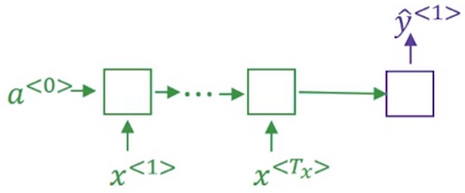4. 贪婪算法只会挑出最可能的那个单词，然后继续，然而 集束搜索算法 会考虑多个结果， 集束搜索算法 会有一个参数B， 叫做 集束宽(beam width) 在这个例子中，设置集束宽(beam width) 为3 这表明其会一次性考虑三个候选单词。然后 集束搜索算法 会把结果存到计算机内存中，以便后面尝试使用这三个词。
5. 过程是先将整个待翻译的句子输入到 绿色 的编码网络中，然后使用 紫色 的解码网络进行解码，结果是一个1W维的向量，用来表示第一个英文单词的概率，选择概率最大的 3个(集束宽) 单词存储在内存中。
2. 对于第二个单词，是要在确定第一个单词的情况下进行搜索。

1. 假设第一个单词被设置为 in 或 jane 或 september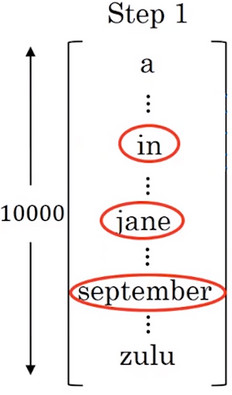2. 将第一个单词 in 作为 解码器 的第一个节点的输出，并且将其作为第二个节点的输入。这样这个网络就能评估第二个词的概率了 $P(y^{<2>}|x,"in")$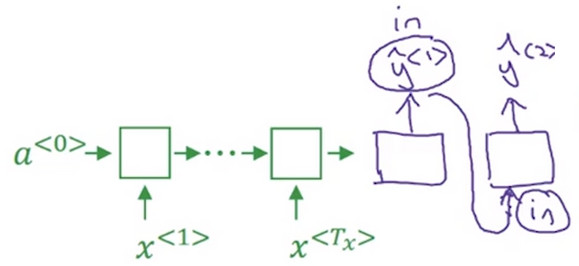3. Note 在第二步中，第一个和第二个单词的联合概率是关心的重点即$P(y^{<1>},y^{<2>}|x)$,根据概率公式，有:$P(y^{<1>},y^{<2>}|x)=p(y^{<1>}|x)P(y^{<2>}|x,y^{<1>})$.
4. 同样，对第一个翻译结果的其他候选词("jane, september")进行如上操作.
5. 由于使用的 集束宽 为3 ，并且词汇表中单词的数量为 1W, 所以最终会有3W(集束宽 * 词典中词汇总量)个可能的结果
6. 再从3W个结果中挑选出3(集束宽)个概率最大的结果
• Note 对于第二个单词的挑选，使用三(集束宽)个不同的网络，因为每个网络的 $y^{<1>}$ 不同。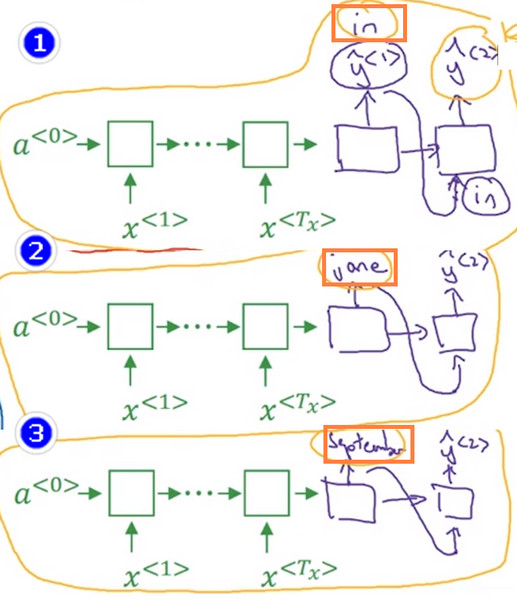3. 保存已经挑选出的前两个单词与在输入为x的条件下，前两个单词的概率，同上述操作挑选出第三个词：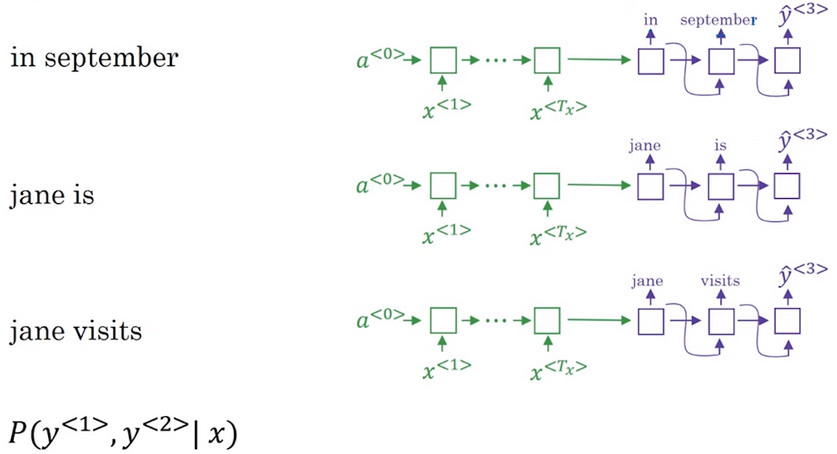4. 集束搜索通过这种方法每次找到一个词，最终 得到 Jane visits africa in september 这个句子终止在句尾符号

#### 取log值

1. 集束搜索的目的是最大化下式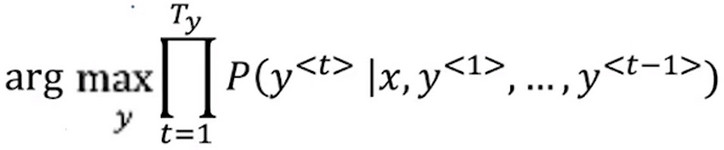$P(y^{<1>}y^{<2>}...y^{T_{y}})=P(y^{<1>}|x)P(y^{<2>}|x,y^{<1>})...P(y^{T_{y}}|x,y^{<1>}...,y^{T_{y}-1})$

1. 但是$P(y^{<1>}|x)P(y^{<2>}|x,y^{<1>})...P(y^{<T_{y}>}|x,y^{<1>}...,y^{<T_{y}-1>})$这个乘积式中的因子都是小数，其乘积会是一个十分小的数，会造成 数值下溢(numerical underflow)
2. 为了解决这个问题 ，将最大化的乘积式取对数 ，由 logM*N=logM+logN 公式可得，上述需要最大化的乘积式可以转化为: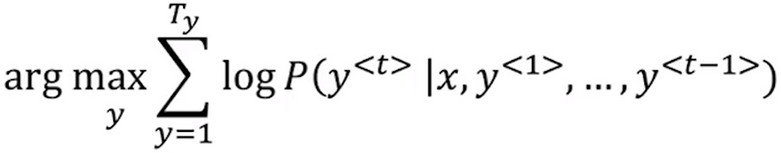#### 归一化

1. 由于$P(y^{<1>}|x)P(y^{<2>}|x,y^{<1>})...P(y^{<T_{y}>}|x,y^{<1>}...,y^{<T_{y}-1>})$乘积式中各个因此都是小数，所以随着翻译句子的增长，P的乘积会越来越小。而 集束搜索 的结果会选取较大的P的乘积式。这样搜索方法会不自然的偏向 更短的翻译输出 因为 短句子 的概率是由更少的小于1的数字乘积得到的。而对于乘积的 对数式 ，由于取对数后的结果是负数，要取得更大的概率值，也会偏向于 更短的翻译结果
2. 因此将原先的公式 除以翻译后句子的总长度/翻译后句子总长度的指数(指数小于1)这样很明显的减少了对输出长的结果的惩罚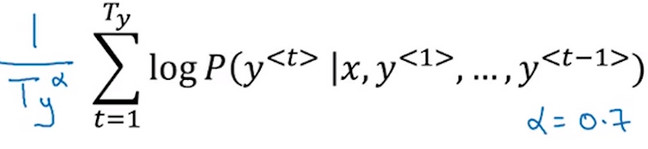#### 束宽选择 Beam width B

• 束宽B越大，你考虑的选择越多，找到的句子可能越好，但是B越大，算法的计算代价越大，程序运行的也会相对较慢，因为要把更多可能的选择保存下来。

• 束宽B越小，需要考虑的选择越少，内存占用小，程序运行越快，但是效果没有那么好。

• 普通时候B一般选择10，工业界上也可以选择100，科研任务中需要得到最好的结果，也有将B设置为1000或3000的时候。

• Note 相对于 深度优先搜索广度优先搜索 等算法来说，束搜索运行的更快但是不能包含保证一定能找到arg max的准确的最大值

• 束搜索算法是一种 近似搜索算法(approximate search algorithm) , 也被称为 heuristic search algorithm 启发式搜索算法 ， 其不总是输出可能性最大的句子 ， 它仅记录着B为前3或者10或者100种可能。 所以束搜索方法也会出现错误。 本节将使用 误差分析 的方法对 束搜索(beam search) 进行改进，发现到底是束搜索方法出现了问题还是构造的RNN模型出现了问题导致整个系统的失效。
• 例句 Jane visite l'Afrique en septembre ,验证集中人工翻译的正确答案为 Jane visits Africa in September 将人工翻译的结果标记为 $y^{ * }$ ,使用训练完成的机器翻译模型翻译为 Jane visited Africa last September 并将其标记为 $\hat{y}$
• 当然，机器翻译的结果不能算是好的翻译，其中机器翻译可以被分成两个部分 编码器与解码器束搜索B 。必须有方法判断出是两部分中的哪部分的问题，导致翻译系统不能很好的工作。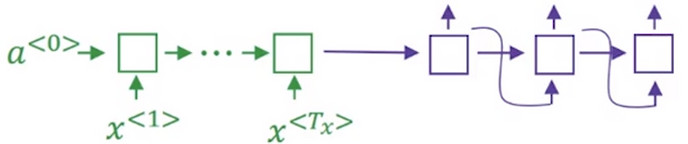• 增大束宽B 意味着在选择单词时有更好的选择 ，但是一味的增大束宽B也会带来不好的结果。
• RNN的功能是计算P(y|x),所以可以通过比较 $P(y^{ * }|x)$$P(\hat{y}|x)$ 的值的大小来判断RNN和束搜索方法的好坏。

#### $P(y^{ * }|x) \ge P(\hat{y}|x)$

• 束搜索方法选择了 $\hat{y}$ , RNN计算P(y|x), 而束搜索方法就是找到了 $\hat{y}$ ，使得P(y|x)达到最大。所以此时能够判断 束搜索方法不能提供一个能是P(y|x)达到最大的y值

#### $P(y^{ * }|x) \le P(\hat{y}|x)$

• 在例子中设定 $y^{ * }$ 是比 $\hat{y}$ 更好的翻译结果，不过根据RNN的概率计算 $P(y^{ * }|x) \le P(\hat{y}|x)$ 这与P(x|y)的实际定义不符合，RNN应该判断更好的翻译结果具有更高的P(x|y)值，所以此时可以认为是RNN模型出了问题，不能对P(x|y)做出有效的判断。

#### 总结

• 通过这个过程，你就能执行误差分析得出束搜索算法和RNN模型出错的比例是多少。可以通过对开发集中每一个错误例子(即翻译比人工差的情况)尝试确定这些错误，是搜索算法还是RNN模型出错。并且通过这个过程可以发现其中哪个对翻译出错的影响更大。并且只有当发现是束搜索算法引发了大量错误时，才可以决定增大集束宽度B。如果是发现RNN出了问题，你可以进行更深层次的分析来决定是需要增加正则化还是获取更多的训练数据或是尝试一个不同的网络结构
posted @ 2018-08-31 15:22  WUST许志伟  阅读(803)  评论(0编辑  收藏